# Excel TODAY function to insert today's date and more

The tutorial shows how to insert today's date in your worksheets and demonstrates a few other uses of TODAY function in Excel.

You want to put today's date in some cell? Or you wish to highlight the current date in your Excel calendar? Or you'd like to find the date closest to today? All this can be done by using the Excel TODAY function and this tutorial will teach you how.

## Excel TODAY function - syntax and basic uses

The TODAY function in Excel does exactly what its name indicates - returns today's date.

The syntax of the TODAY function is as simple as it could possible be - it does not have any arguments at all. Whenever you need to insert today's date in Excel, just enter the following formula in a cell:

`=TODAY()`

You can format the value returned by TODAY in any built-in or custom date format. For example, this way: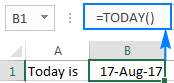### 4 things you should know about TODAY in Excel

1. TODAY() is a volatile function, which means that it updates itself continuously every time a worksheet is opened or changed.
2. If a TODAY formula does not update automatically, most likely automatic recalculation is turned off in your workbook. To turn it on again, go to the Formulas tab > Calculation Options, and select Automatic.
3. To enter today's date in Excel as a static unchangeable value, use these keyboard shortcuts.
4. If you want to insert current date and time, use the NOW() function instead of TODAY().

## How to insert today's date in Excel

There are two ways to enter the current date in Excel - a formula and shortcut. Which one to use depends on whether you want a static or dynamic value.

### Excel formula for today's date

The value returned by the TODAY function updates automatically, so the below formula is useful if you want the worksheet to always display the current date, regardless of when you open it.

`=TODAY()`

To clarify what kind of date that is, you can concatenate TODAY() with some explanatory text, for example:

`=CONCATENATE("Today is ",TEXT(TODAY(), "mmmm dd, yyyy"))`

Because in the internal Excel system dates and times are stored as numbers, concatenating text with the TODAY() formula directly would result in a meaningless string like "Today is 42965". To avoid this, we nest Excel's TODAY function within the TEXT function to display the date in the desired format.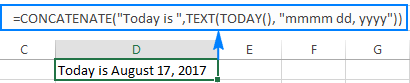### Shortcuts to get today's date in Excel

The inset today's date as an unchangeable timestamp that won't automatically update the next day, use one of the following keyboard shortcuts:

• To inset today's date:  Ctrl + ;
• To insert the current time: Ctrl + Shift + ;
• To enter the current date and time: Ctrl + ; then Space and then Ctrl + Shift + ;

## How to use TODAY function in Excel - formula examples

As you already know, the main purpose of the Excel TODAY function is to get today's date. Aside from that, you can use TODAY() in combination with other functions to perform more complex calculations based on the current date. Below you will find a few examples of such formulas.

### Add or subtract days to/from today's date

To add or subtract a specific number of days to/from the current date, use a simple arithmetic operation of addition or subtraction, respectively.

For example, to add 7 days to todays' date, use this formula:

=TODAY()+7

To subtract 7 days from the current date, go with this one:

=TODAY()-7

To exclude weekends (Saturday and Sunday) from your calculations, nest the TODAY function within WORKDAY that deals with weekdays only:

To add 7 workdays to today's date:

=WORKDAY(TODAY(), 7)

To subtract 7 workdays from today's date:

=WORKDAY(TODAY(), -7)

The following screenshot shows the results: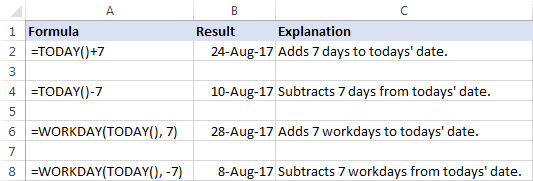Tip. To display the calculated date correctly, set the formula cell's format to Date.

### Get the number of days before or after a certain date

To calculate how many days are left before some data, subtract today's date from the future date you are counting toward:

date-TODAY()

The date can be supplied directly to a formula in the format that Excel can understand, or by using the DATE function, or as a reference to the cell containing the date.

For example, to find out how many days are left till December 31, 2017, use one of these formulas:

`=A2-TODAY()`

`=DATE(2017,12,31)-TODAY()`

`="12/31/2017"-TODAY()`

All three formulas tell us that at the moment of writing (August 17, 2017), 136 days were left till the end of the year 2017: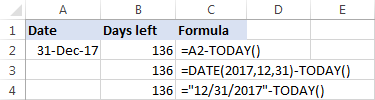To calculate the number of days since a certain date, subtract the past date from today's date:

TODAY()-date

For example, to find out how many days have passed since January 1, 2017, use one of these formulas:

`=TODAY()-A2`

`=TODAY()-DATE(2017,1,1)`

`=TODAY()-"1/1/2017"`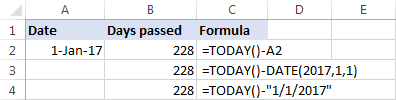Tip. If the result is not displayed correct, be sure to apply the General format to the formula cell.

### Calculate months since or before a certain date

To get the number of months between today and a past date, use the DATEDIF(start_date, end_date, unit) function with the past date in the start_date argument, TODAY() as end_date and "m" unit denoting months:

DATEDIF(past_ date, TODAY(),"m")

To get the number of months between today and a future date, swap the start_date and end_date arguments:

DATEDIF(TODAY(), future-date,"m")

With the date of interest in cell A4, use the following formulas to calculate time difference in the number of complete months:

To calculate months since a certain date in the past:

`=DATEDIF(A4,TODAY(),"m")`

To calculate months before a certain date in the future:

`=DATEDIF(TODAY(),A4,"m")`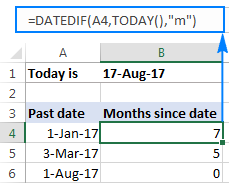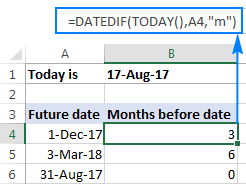### Calculate years since / before a certain date

The formulas to calculate years based on today's date are similar to the ones discussed in the above example. The difference is that you use "y" unit to get the number of complete years between today and another data:

To calculate years since a past date:

DATEDIF(past_ date, TODAY(),"y")

To calculate years before a future date:

DATEDIF(TODAY(), future-date,"y")

Assuming the past/future date is in cell A4, you should be good with using these formulas:

The number of complete years since date:

`=DATEDIF(A4,TODAY(),"y")`

The number of complete years before date:

`=DATEDIF(TODAY(),A4,"y")`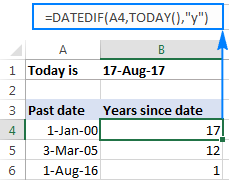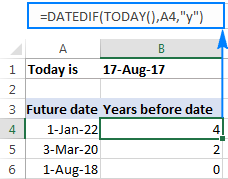### Get age from birthdate

If you know someone's year of birth, you can subtract that year from the current year to find the person's age:

YEAR( TODAY())-year_of_birth

For example, if the person was born in 2000, you use the following formula to get his/her age:

`=YEAR( TODAY())-2000`

Or, you can enter the year of birth in a separate cell and reference that cell in your formula: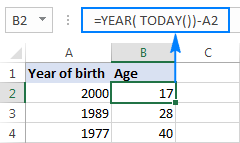You can learn a few other age calculation formulas in this tutorial: How to get age from date of birth in Excel.

### Find the nearest date to today

If you are curious to know which date in a list is closest to today's date, use one of the following array formulas to find it out.

#### Get a past date closest to today

To find a past date nearest to the current date, first "filter out" the dates greater than today, and then use the MAX function to get the largest date among the remaining ones:

MAX(IF(range < TODAY(), range))

With the dates in cells A2 to A10, the formula would go as follows:

`=MAX(IF(\$A\$2:\$A\$10 < TODAY(), \$A\$2:\$A\$10))`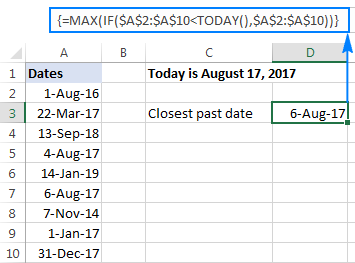#### Get a future date closest to today

To find the nearest future date, identify the dates that are greater than today, and then use the MIN function to get the smallest date among them:

MIN(IF(range >TODAY(), range))

For our sample data set, we'd use this formula:

`=MIN(IF(\$A\$2:\$A\$10 > TODAY(), \$A\$2:\$A\$10))`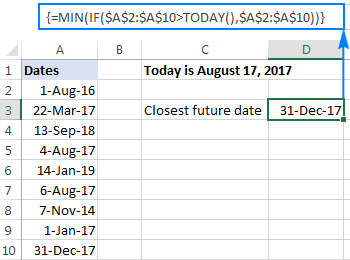#### Get any date closest to today

To get the nearest date in a mixed list of past and future dates, use the classic INDEX MATCH formula with a couple of modifications:

INDEX(range, MATCH(MIN(ABS(range - TODAY())), ABS(range - TODAY()), 0))

Here's how this generic formula works:

• MIN(ABS(range -TODAY())) part. First, you subtract today's date from each date in the range of dates. Then, the ABS function returns the differences as absolute values without regard to their sign. The MIN finds the minimal value, which goes to INDEX MATCH as the lookup value.
• ABS(range -TODAY()) part. You subtract today's date from the range of dates and return an array of absolute values. This array is where INDEX MATCH searches for the lookup value.

In this example, the formula takes the following shape:

`=INDEX(\$A\$2:\$A\$10, MATCH(MIN(ABS(\$A\$2:\$A\$10 - TODAY())), ABS(\$A\$2:\$A\$10 - TODAY()), 0))`

The screenshot below shows the result: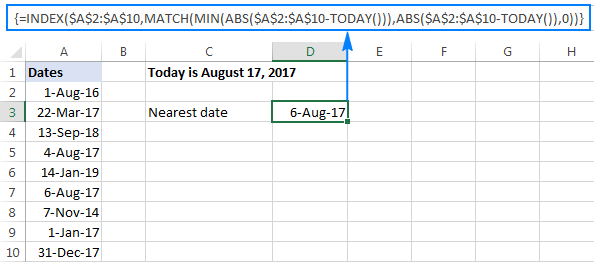Note. All three formulas to get the nearest date are array formulas, so they should be completed by pressing Ctrl + Shift + Enter.

### How to highlight today's date in Excel

When working with a long list of dates or designing your own calendar in Excel, you may want to have the current date highlighted. To have it done, create a conditional formatting rule with this formula:

`=B2=TODAY()`

Where B2 is the left-top-most cell of the range to which the rule applies.

The result may look something similar to this: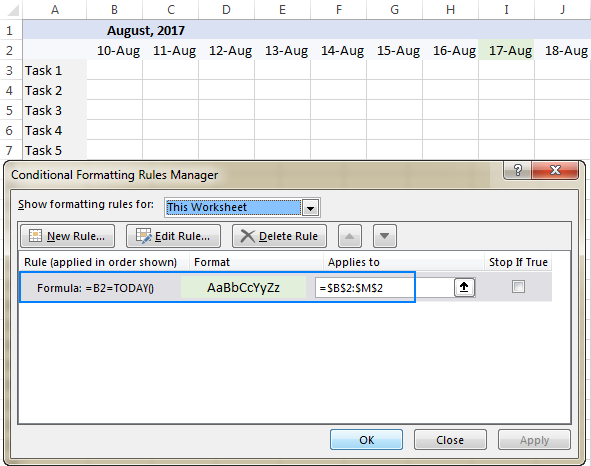The detailed steps to set up a conditional formatting rule can be found here: How to create a conditional formatting rule based on formula.

To have a closer look at the formulas discussed in this tutorial, you are welcome to download our Excel Today's Date workbook.

### More examples of TODAY formula in Excel

For more examples of using the TODAY function in Excel, please check out the following tutorials:

### 46 Responses to "Excel TODAY function to insert today's date and more"

1.STEVE says:

Can you help please? I have a spreadsheet on which I want to do the following: if I enter a number in a cell in Col A I want the adjacent cell in Col B to return a date which is today plus 21 days. If no number is entered in a cell in Col A I want the adjacent cell in Col B to remain blank. So:
Col A Col B
2 today's date + 21 days is returned

3 today's date + 21 days is returned
1 today's date + 21 days is returned

•Svetlana Cheusheva (Ablebits.com Team) says:

Hi Steve,

Supposing your data begins in row 2, you enter the following formula in B2, and then copy it down the column:
=IF(ISNUMBER(A2), TODAY()+21, "")

•Madhu says:

Thanks you much , its more useful.

•Vignesh says:

Can we get a trend of values based on today fuction.I am using the todays value to divide expenses to get the average expenses daily

2.Doug says:

Steve:
Enter this into cell B2 adjacent to cell A2
=IF(ISNUMBER(A2),(TODAY()+21),"")
then copy the formula down the B column.

3.venus says:

THANK YOU. A million times, THANK YOU!!!

4.Louis Villemaire says:

Hello Svetlana, where you're from, Ukraine or Russia? :)
Nice job!
Thanks you dear...

•Svetlana Cheusheva (Ablebits.com Team) says:

Thank you, Louis. I'm from Belarus :)

•Anwar Ali says:

Thanks its help me a lot

5.Linson says:

HI Steve
can you suggest me a formula, where i need current date in date coloum if i make any change in that particular Row.

6-May-18 . Linson N/A N/A 20-Jun-17 20-Jun-17

so if make any change in any details by previous date (6-May-18) should become current date.

•Doug says:

Linson:
I believe if you want a cell to update based on change to another cell it requires some code. In this case I think you could use some VBA, but VBA is not in this blog's frame. If you wanted to tackle this you could google "excel update cell if another cell changes".

6.Mandi says:

I have a column of dates with some empty cells - how can i fill in all the empty cells with today's DATE?

•Doug says:

Mandi:
TODAY() will give you today's date. If you enter this function in a cell, the cell will always display the current date.

7.NgounOung says:

Dear Everyone

May anyone can help me please
i would like to have a function that when we key in today it will be show date today this date will be not change since we already move to next day..
or When we key in on one cell date today will show automatically.

Thank you so much

8.R says:

Hi,

How do you add the time to the Excel formula for today's date? Using the formula,

=CONCATENATE("Today is: ",TEXT(TODAY(), "dd mmmm yyyy hh:mm:ss"))

I get "Today is: 25 October 2018 00:00:00" -- and not the current time, no matter what time it is. Even after reading many, many, websites on this topic, I am still confused (or rather, more confused), and I'm not really sure how the time stamp can still be added.

Thanks and much appreciated,
R

•J says:

Use NOW(), not TODAY(). TODAY() is only the date, NOW() includes the time.

9.Jim K says:

How do I auto fill just the current Month?Like December, January or February in a cell every time I open the document? I am making forms that I only want the month not today's date

•Doug says:

Hello, Jim:
To enter the current month in a cell enter this formula in the cell:
=TEXT(TODAY(),"mmmm")

10.Andy T says:

Guys,
I have all the dates for the year in column A starting in cell A2 from 01-Jan-19 through to 31-Dec-19. I used your guidance to create a rule to highlight the current day (thanks). I would like to create a rule so that all of the days that are now past would be in a gray text colour, whereas the current date and future dates remain in a black font colour. Any advice much appreciated.

•Jonathan says:

=C1:C99<TODAY()-30

this has highlighted for me the dates that are 30 days past from today. I use this in my outreach sheet to keep relationships current

11.Audra says:

I have the TODAY function in cell A1. I want cell G9 to update to the previous month/year. Ex. Today's date is 1/11/2019 in cell A1 so I want G9 to show December 2018. And then when A1 shows 2/1/19, I want G9 to show January 2019. Does a function and/or condition exist for this?

12.Anwar Ali says:

@ Svetlana Cheusheva: Thanks for saving a lots of time.

13.Bob says:

I have a list of events in column A with completion dates in column B. I use this formula to find out how many events have not been completed in one (1) year:
=COUNTIF(B2:B202,"<2/4/2018")
I manually put in the date as today's month and day and last year. Is there a way I can have Excel do that so it is always up to date without me having to manually entering the date? I tried this but it does not work: =COUNTIF(C2:C202,"<TODAY()-365")

Thank you,
Bob

14.Hector says:

Hi Svetlana,
Was wondering if there is a way to have excel change the year of a date entered. For example I enter 5/29 (and I have formatted cells to the 03/14/01 format), so excel returns 05/29/19. But I want a formula so that it returns 05/29/18. So same date just for last year?
Thank you very much for your help. Love your name by the way.

15.IAN says:

Can somebody help me how to get the date of loyalty of employee in 10 years, example supposing he/she was hired March 03, 2008 then he got received loyalty award at March 03, 2018. This date will show in cell. Then if he/she 15 years of received he/she will received again loyalty award of 5 years. The date will also show when he/she will received that award. Thanks in advance. This is a great help for me! God bLess :)

16.Aleem says:

Hello,
if i enter in cell name of the task, the other cell need to show the date.

17.Daniel says:

Cell A2 =TODAY(), the data in cells B2:G2 fail to move down 1 row to A3 when A2's date is updated. I would also like the formatting from below to automatically be applied to B2:G2 as the new row is created. Thank you.

18.Aharon Smith says:

Hello, thanks for the post! It is very informative.
I am working on a budget spreadsheet and I am trying to find the correct syntax for an IF ELSE formula for Excel. Here is my formula:
IF(TODAY()>06/01/2019,G10-G11,"Not EOM")
The goal is to have the program give the difference between income (G10) and expenses (G11) AFTER the end of the month. It doesn't seem to be recognizing the ">" sign. For it gives G10-G11. Can you help? Thanks!

•Dean says:

Not sure if your question was ever answered, but you could consider using the number format of the date, in your case 43471. This is how excel uses dates in calculations, and so may aid you.
i.e.
IF(TODAY()>43471,G10-G11,"Not EOM")

Cheers,
D

19.Seb says:

Hopefully someone will be able to tell me what i am doing wrong here. I have created a seperate worksheet that shows data for each day of the year as either "a" or "u". I'm wanting to create a formula that will count the number of occurences of "u" in the next 7 days and the next 30 days. Each formula I attempt throws an error as i can't figure a way to create a date range using todays date as the start point.
I started with the below for counting out to 7 days
=COUNTIFS('Calendar'!\$2:\$2,TODAY()+7,'Response'!3:3,"U") or =COUNTIFS('Calendar'!\$2:\$2,TODAY():TODAY()+7,'Response'!3:3,"U")

Hopefully someone can help me. Thanks!!

20.Gagan Bhatia says:

If have =+TODAY()+1 in cell that will give me tomorrow date, I want it will stop automatically when month will be over on 30th or 31st

21.rICHARD says:

Hi

If I input on a cell number '1' then the current date will show, if '0' the cell leave blank.

Thanks for help

22.Michael Halpner says:

Hello- can really use help with this.....I have a list of assets in one column with just a number representing the value of how old each asset is.....I need a formula for another column- YEAR BUILT- so I need a YEAR formula that I can combine with TODAY()......I just can't figure out how to do it....I need to use TODAY(), because this would be an ongoing list

23.Megan says:

Hi I am using the TODAY formula in a roster to highlight the current date on a roster however it is highlighting the incorrect date, the date highlighted is two days ahead of the correct date.

24.abhi says:

how to freeze =now() or =today()? It must not change when we entered anywhere in cells
=if(A2="",today(),"1")

25.WAS INSTITTUTE says:

I WOULD TO HAVE ACCESS TO POWER PIVOT,POWER QUERRY COURSE,POWER B TUTORIAL

26.Ravi says:

Hi,
I need like this formula,
D36 is 15-Aug-2019
=IF(AND(D36>TODAY(), AND((=TODAY()-D36)>=0, (=TODAY()-D36)<=10))),"Inside","Outside")
When i use this one getting error, so please explain where i did mistake?
thank you

27.Vi says:

Hi,
I'm always looking at prior day and getting it =TODAY()-1. How can I get the Fridays date on Monday by using TODAY() formula?

28.Mgd says:

Hello, can anyone please help. I have a list of renewal dates and I’m looking for an excel formula which will highlight in yellow, the dates which are less than 1 month from today. Thank you

29.Phil says:

I'd like excel to calulate the time between two dates to show how long someone was on a waiting list but when the second date is blank I'd like excel to calculate using today's date. I can do those two functions separately but not sure how to do them both together?

30.Carmen says:

I am attempting to use the formula as indicated above, however the result is displayed as #VALUE!
I am using the following string:
=MIN(IF(\$E\$5:\$E\$9>TODAY(),\$E\$5:\$E\$9))
Any idea where I am going wrong?

31.Zack says:

Hi, how to make a formula that counts the items that have yesterday and today's date?

32.Chanu says:

hello experts,
is there any formula to get the monthly date from cell A1 to A30/31?
for example:
A1= 1/10/2019
A2=2/10/2019
.
A31 =31/10/2019

33.Aneesa says:

How do I do an age analysis on Excel?
Created Date Time Age Analyse (TODAY)
21/06/2019 12:19

34.Meher says:

=Today() default format(mm/dd/yyy) can be changed to dd/mm/yyyy?

60+ professional tools for Excel